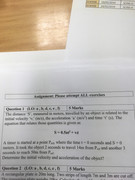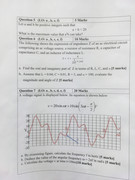User Name Remember Me? Password

 Math General Math Forum - For general math related discussion and news

 February 13th, 2019, 11:44 AM #1 Newbie   Joined: Feb 2019 From: Scotland Posts: 4 Thanks: 0 Help with assignment Can anyone help with these assignment questions? I don’t know where to start and need a push in the right direction.Last edited by skipjack; February 16th, 2019 at 06:35 AM.February 13th, 2019, 02:47 PM #2 Math Team   Joined: Oct 2011 From: Ottawa Ontario, Canada Posts: 14,597 Thanks: 1039 What do you expect? No math teacher?February 13th, 2019, 03:05 PM #3 Senior Member   Joined: Dec 2015 From: somewhere Posts: 746 Thanks: 100 $\displaystyle a+b=20 \;$ a,b positive integers , max$\displaystyle (a^2 b )=?$ $\displaystyle a^2 b=20a^2 -a^3 \;$ now by derivatives $\displaystyle 40a-3a^2=0 \;$ gives $\displaystyle a=40/3$ , but it must be an integer so choose the nearest integer less than $\displaystyle 40/3$ which is $\displaystyle 13$ and $\displaystyle b=7$ . The maximal value $\displaystyle a^2 b$ can take is $\displaystyle 13^2 \cdot 7 =1183$ . Thanks from jks, topsquark and Gersrus71February 13th, 2019, 06:13 PM   #4
Senior Member

Joined: Sep 2016
From: USA

Posts: 670
Thanks: 440

Math Focus: Dynamical systems, analytic function theory, numerics
Quote:
 Originally Posted by idontknowbut it must be an integer so choose the nearest integer less than $\displaystyle 40/3$ which is $\displaystyle 13$ and $\displaystyle b=7$ .
Everything up to here is fine but this reasoning is completely invalid. There are countless counterexamples and the subject of integer linear programming exists because you can't do optimization on the integers by doing optimization on the reals and then looking "nearby" for an integer solution.February 13th, 2019, 11:34 PM   #5
Newbie

Joined: Feb 2019
From: Scotland

Posts: 4
Thanks: 0

Quote:
 Originally Posted by DenisWhat do you expect? No math teacher?
I’m doing distance learning so no I don’t have a teacher as such.
I haven’t done Maths since school so I’m struggling a bit so was just looking for some pointersFebruary 14th, 2019, 04:29 PM   #6
Global Moderator

Joined: May 2007

Posts: 6,835
Thanks: 733

Quote:
 Originally Posted by SDKEverything up to here is fine but this reasoning is completely invalid. There are countless counterexamples and the subject of integer linear programming exists because you can't do optimization on the integers by doing optimization on the reals and then looking "nearby" for an integer solution.
Although your statement is true in general, the approach works in this case, where it is maximizing a polynomial.February 14th, 2019, 05:20 PM   #7
Senior Member

Joined: Sep 2016
From: USA

Posts: 670
Thanks: 440

Math Focus: Dynamical systems, analytic function theory, numerics
Quote:
 Originally Posted by mathmanAlthough your statement is true in general, the approach works in this case, where it is maximizing a polynomial.
I'm aware that in this case it happens to give the correct answer. My comment was intended to point out the flaw in logic. If a student turned in something like that with the "right" answer and that explanation it would be graded pretty harshly.

Also, it has nothing to do with being a polynomial. One proof that it works in this example easily follows from the AM/GM inequality. So more generally it will work for monomials. I can't see any structure that is more general which would make this still work. It is definitely false for polynomials in general. Here is a simple counterexample.

$p(x) = -\frac{118}{75}x^4 + \frac{758}{75}x^3 - \frac{3121}{150}x^2 + \frac{2141}{150}x$

is a polynomial with rational coefficients even. Its easy to check that if you maximize this on $[0,4]$ the maximum is at $x = 2.5$. But $p(2) = p(3) = 1$ are not the maximum over the integers since $p(1) = 2$. This example wasn't particularly hard to generate.

Last edited by SDK; February 14th, 2019 at 05:24 PM.February 15th, 2019, 02:55 AM #8 Senior Member   Joined: Dec 2015 From: somewhere Posts: 746 Thanks: 100 The derivatives are not a method for integer variables . But using the graph and a bit of brute force is easy to find the maxima and minima. If the local extremum exists , then one of the ineger parts like floor(x) or ceil(x) will be the point of extremum for integers , if the integers are in the domain . Also knowing whether the function is increasing or decreasing in interval we want . Last edited by idontknow; February 15th, 2019 at 03:28 AM.February 15th, 2019, 05:00 AM   #9
Senior Member

Joined: Sep 2016
From: USA

Posts: 670
Thanks: 440

Math Focus: Dynamical systems, analytic function theory, numerics
Quote:
 Originally Posted by idontknowThe derivatives are not a method for integer variables. But using the graph and a bit of brute force is easy to find the maxima and minima. If the local extremum exists , then one of the ineger parts like floor(x) or ceil(x) will be the point of extremum for integers , if the integers are in the domain . Also knowing whether the function is increasing or decreasing in interval we want .
I'm confused. First you agree that derivatives should not be used for integer optimization, but then you say it works. To address your claims specifically:
1. "The derivatives are not a method for integer variables."

I agree

2. "But using the graph and a bit of brute force is easy to find the maxima and minima."

I'm not sure how the graph helps here. The graph of what function? How is determining the graph easier than determining where max/min values are? I agree that for this problem the "correct" answer is to brute force search the integers in the domain.

3. "If the local extremum exists , then one of the ineger parts like floor(x) or ceil(x) will be the point of extremum for integers , if the integers are in the domain ."

This is false which was my entire point. I have provided a counterexample above. What is worse is that this isn't even "usually" true in practice. Hence the reason for point (1). You shouldn't use calculus to optimize over integers.

4. "Also knowing whether the function is increasing or decreasing in interval we want ."

Determining where a function is increasing/decreasing is no easier than optimizing it. In fact, they are equivalent at best since obviously if you know whether the function is increasing/decreasing at every point, then you must know where it attains max/min values. But typically this question is even harder. Moreover, you would still need to know where it takes integral values which is even harder than that.February 15th, 2019, 05:07 AM #10 Newbie   Joined: Feb 2019 From: Scotland Posts: 4 Thanks: 0 That’s all good stuff lads but none of it really helps with any of my problems... well apart from 1. Does anyone have any pointers to helping me out with any of the other stuff?Tags assignmentThread ToolsShow Printable VersionEmail this Page Display ModesLinear ModeSwitch to Hybrid ModeSwitch to Threaded ModeSimilar Threads Thread Thread Starter Forum Replies Last Post lilshrekboye29 Calculus 2 February 11th, 2019 01:01 PM mayak201 Physics 5 April 8th, 2018 01:33 PM illidan5 Number Theory 5 March 24th, 2016 04:31 AM LMW Algebra 1 November 20th, 2012 02:46 PM ron Calculus 1 October 8th, 2011 09:36 AM

 Contact - Home - Forums - Cryptocurrency Forum - Top